## 19 February 2016

### MS188-110C, Appendix C/D scrambler

Both the High Speed Waveforms (HSWF) and Wide Band HF Radio waveforms (WBHF), described in the Appendices "C" and "D" from the standard MS188-110C, use the same scrambler. Modems operating over multiple discrete channels (Appendix F) also use this same scrambler since they use the waveforms from Appendix C. The scrambling sequence generator polynomial is:
x^9 + x^4 + 1
and is initialized to 00000001 at the start of each data frame, i.e. each 256 transmitted symbols (data block lenght is the same in both the two waveforms families). The length of the scrambling sequence is 511 bitscomputed as the maximal number of its states excluding the all-zeroes state (2^9 -1). For a 256 symbol data block with 4 bits per symbol, this means that the scrambling sequence will be repeated about 2 times, while for 6 bits per symbol just slightly more than 3 times, although in terms of symbols there will be no repetition (pseudo-random generator). In other words, the scrambler is designed to not have auto-correlation property, contrary to what happens for the MS188-110A scrambler, as we saw here, where the scrambler produces a periodic pattern 160 transmit symbols (480 bits, since the PSK-8 modulation) in length that at certain data rate speeds affects the value of ACF.
I do not want reinvent the wheel here but only practice of analysis, so I just looked for a confirmation of this behavior (and described below) by analyzing the bitstream produced by a software-scrambler that I wrote in Lua language for both PSK-8 and QAM-16 modulations, in the latter case I also examined a real-world QAM-16 signal to verify the lack of possible signs/repetitions caused by the scrambler.

PSK-8 modulation
For PSK-8 data symbols (3200 bps and 4800 bps), the scrambling shall be carried out taking the modulo 8 sum of the numerical value of the binary triplet consisting of the last (rightmost) three bits in the shift register, and the symbol number.  A block diagram of the scrambling sequence generator is shown in pic. 1, in this illustration, three output bits are shown: this is the case for PSK waveforms.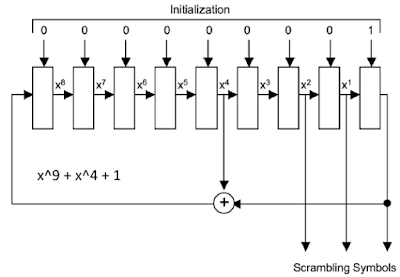Pic. 1
After each data symbol is scrambled, the generator shall be iterated (shifted) the required number of times to produce all new bits for use in scrambling the next symbol, so will be 3 iterations for PSK-8. Since the generator is iterated after the bits are use, the first data symbol of every data block (256 symbols) shall, therefore, be scrambled by the appropriate number of bits from the initialization value of 00000001.pic. 2
The sw-scrambler writes the scrambled symbols, ready to be sent to the PSK modulator, to the "scrambler-output.txt" file. Examining this bitstream (pic. 2) a 768 bits period is revealed and this lenght is exactly the lenght of the 256 PSK-8 symbols data block: there is no evidence of 511 bits cycle due to the scrambler.

QAM-16 modulation
The data symbols for QAM modulations shall be scrambled by using an exclusive or (XOR) operation: sequentially, the data bits forming each symbol (4 for QAM-16, 5 for QAM-32, 6 for QAM-64 and 8 for QAM-256) shall be XORed with an equal number of bits from the scrambling sequence (pic. 3).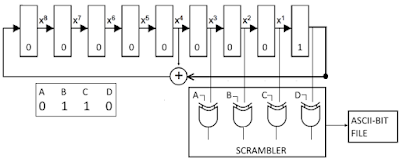pic. 3 - scrambler for QAM-16 modulation
After each data symbol is scrambled, the generator shall be iterated 4 times to produce all new bits for use in scrambling the next symbol. As said, since the generator is iterated after the bits are use, the first data symbol of every data frame shall, therefore, be scrambled by the appropriate number of bits from the initialization value of 00000001. I used a constant data symbol value (0110) just to highlight the behavior of the scrambler.pic. 4 - running the sw-scrambler for QAM-16 modulation
The sw-scrambler writes the scrambled symbols to the "QAM-16-scrambler-output.txt" file. Examining this 5000 symbols bitstream (pic. 5) a 1024 bits period is revealed. As in the case of PSK-8 scrambler, this lenght is exactly the lenght of the 256 QAM-16 symbols data block: also in this case there is no evidence of 511 bits cycle due to the scrambler.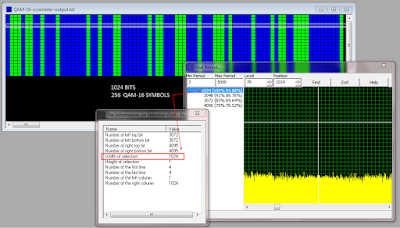pic. 5
real-world QAM-16 signal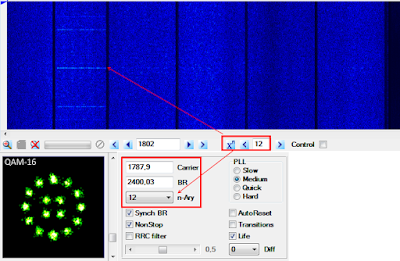pic. 6a - real-world MS188-110C App.D signalpic. 6b - MS188-110C App.D, QAM-16 ACF
As expected, the ACF returns a 120ms period (pic. 6) that makes 288 symbols length frame at 2400 Baud. Since the data block for QAM-16 modulation is always 256 symbols, it follows that mini-probes are 32 symbols lenght (waveform n.8 from Appendix D TABLE D- XI):

After demodulating the QAM-16 signal with SA, it was then converted into an ASCII-bit file by using a simple HEX2BIN converter also written in Lua: the output file was then analyzed using a bit-flow processor tool.
The analysis of the bitstream reveals a strong 1152 bits period that is exactly what is expected for the 4-bits symbols WBHF waveform (pic.7):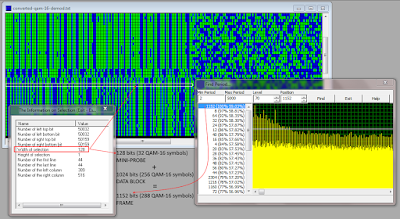pic. 7
data-block: 256 symbols = 1024 bits
mini-probe: 32 symbols = 128 bits
frame (data-block + mini-probe): 288 symbols = 1152 bits
and in terms of symbols, there are no repetition caused by the scrambler (no auto-correlation property).

The same scrambling sequence generator polynomial x^9 + x^4 + 1 is also used in STANAG-4285 waveform (see Annex-A to STANAG-4285) but with a different inizialization vector (see the picture below and pic. 1):
and the results are obviously the same, the bit flow processor only detects the expected 768 bits period: